You may also likeConsecutive Numbers

An investigation involving adding and subtracting sets of consecutive numbers. Lots to find out, lots to explore.Have You Got It?

Can you explain the strategy for winning this game with any target?Counting Factors

Is there an efficient way to work out how many factors a large number has?

Missing 9s

Age 11 to 14 ShortChallenge Level

Counting 300 places on Sara's list
Between 1 and 100, Sara won't list 9, 19, 29, 39, 49, 59, 69, 79, 89 or 90 - 99.
19 numbers will be missed between 1 and 100, 81 will be listed
100 will be the 81st number
81 numbers will be listed between 101 and 200
200 will be the 162nd number
81 numbers will be listed between 201 and 300
300 will be the 243rd number
301 will be the 244th number
...
308 will be the 251st number
310 will be the 252nd number
...
318 will be the 260th number
320 will be the 261st number
...
328 will be the 269th number
330 will be the 270th number
...
340 will be the 279th number
...
350 will be the 288th number
...
360 will be the 297th number
...
363 will be the 300th number

Counting in blocks with a diagram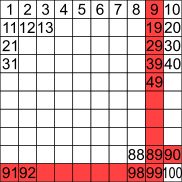Representing the numbers 1 to 100 in a square, the numbers Sara won't list are shown in red.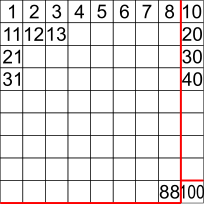The numbers up to 100 on Sara's list make a 9 by 9 square.

81 numbers in this square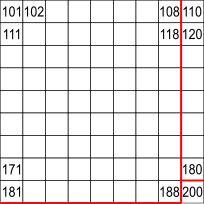The square from 101 to 200 is similar
81 numbers in this square makes 162 altogether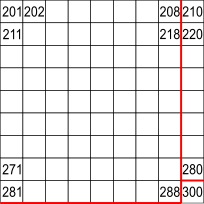The square from 201 to 300 is similar
81 numbers in this square makes 243 altogether
57 more numbers to reach 300Rows from the square from 301 to 400
The rows are all 9 numbers long
9$\times$6 = 54 numbers in this block
3 more numbers to reach 300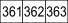300th number is 363

Using base 9
Numbers written in base 9 do not contain any 9s.

In base 9, the numbers 1, 2, 3, 4, 5, 6, 7, 8 are written as usual, but the number '9' is written as 10. Instead of having a ones column and a tens column and a hundreds (=10$^2$) column and so on, base 9 has a ones column and a nines column and an eighty-ones (=9$^2$) column and so on. For example, '18' is written as 20 because it is 2 nines and 0 ones, and '82' is written as 101 because it is 1 eighty-one, 0 nines and 1 one.

To find the 300th number on Sara's list, write 300 in base 9.
3$\times$81 = 243 < 300
4$\times$81 = 324 > 300
There are 3 eighty-ones in 300, remainder 57.

57 = 6$\times$9 + 3,

So 300 = 3$\times$81 + 6$\times$9 + 3
In base 9 it is written 363.

You can find more short problems, arranged by curriculum topic, in our short problems collection.